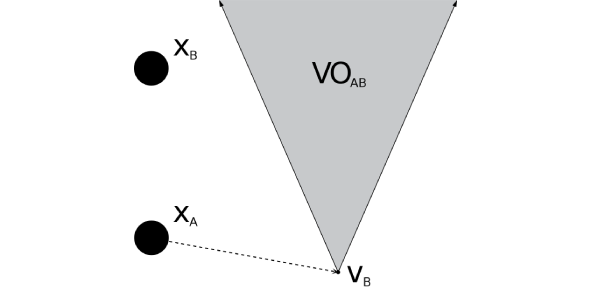# Velocity Equation Test! Trivia Questions Quiz

9 Questions | Total Attempts: 96Settings. What do you know about velocity, and do you think you can pass this quiz? The velocity of an object is the rate of change of its position in a framework of reference and is a act of time. Velocity is equivalent to a specification of an object’s speed and direction of motion. If you would like to discover further about the velocity equation, this is the quiz for you.Back to top